Test: Probability and Statistics - 1

# Test: Probability and Statistics - 1

Test Description

## 20 Questions MCQ Test Topic-wise Tests & Solved Examples for IIT JAM Mathematics | Test: Probability and Statistics - 1

Test: Probability and Statistics - 1 for Mathematics 2023 is part of Topic-wise Tests & Solved Examples for IIT JAM Mathematics preparation. The Test: Probability and Statistics - 1 questions and answers have been prepared according to the Mathematics exam syllabus.The Test: Probability and Statistics - 1 MCQs are made for Mathematics 2023 Exam. Find important definitions, questions, notes, meanings, examples, exercises, MCQs and online tests for Test: Probability and Statistics - 1 below.
Solutions of Test: Probability and Statistics - 1 questions in English are available as part of our Topic-wise Tests & Solved Examples for IIT JAM Mathematics for Mathematics & Test: Probability and Statistics - 1 solutions in Hindi for Topic-wise Tests & Solved Examples for IIT JAM Mathematics course. Download more important topics, notes, lectures and mock test series for Mathematics Exam by signing up for free. Attempt Test: Probability and Statistics - 1 | 20 questions in 60 minutes | Mock test for Mathematics preparation | Free important questions MCQ to study Topic-wise Tests & Solved Examples for IIT JAM Mathematics for Mathematics Exam | Download free PDF with solutions
 1 Crore+ students have signed up on EduRev. Have you?
Test: Probability and Statistics - 1 - Question 1

### Consider the experiment of throwing two fair dice. What is the probability that the sum of the numbers obtained in these dice is even?

Detailed Solution for Test: Probability and Statistics - 1 - Question 1

As sum of two odd numbers and sum of two even numbers is even, so required probability is,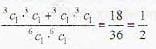Test: Probability and Statistics - 1 - Question 2

### Two dice are rolled until the sum of the numbers appearing on these dice is either 7 or 8. What is the probability that the sum is 7?

Detailed Solution for Test: Probability and Statistics - 1 - Question 2

Sum of the numbers on the faces of t wo dice is 7 in 6 cases and 8 in 5 cases., so by conditional probability’s theorem of Bayes the sum obtained will be 7 has probability,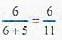Test: Probability and Statistics - 1 - Question 3

### A pair of unbiased dice is rolled together till a sum of either 5 or 7 is obtained. The probability that 5 comes before 7 is.

Detailed Solution for Test: Probability and Statistics - 1 - Question 3

Number of cases for sum ‘5’ = 4
Number of cases for sum ‘7’ = 6
Probability of getting sum 5 in one roll = 4/36 = 1/9
Probability' of getting neither 5 nor 7 in a roll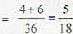⇒ Probability of getting 5 before 7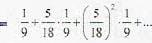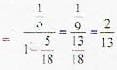Test: Probability and Statistics - 1 - Question 4

A letter is taken at random from the letters of the word ‘STATISTICS ’ and an other letter is taken at random from the letters of the word ‘ASSISTANT’. The probability that, they are the same letter is .

Detailed Solution for Test: Probability and Statistics - 1 - Question 4

The same letter can be either, S,T, I or A.
Probability required will be.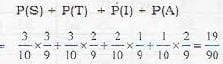Test: Probability and Statistics - 1 - Question 5

A bag contains 6 red and 4 green balls. A fair dice is rolled and a number of balls equal to that appearing on the dice is chosen from the urn at random. The probability that all the balls selected are red is.

Detailed Solution for Test: Probability and Statistics - 1 - Question 5

Required probability =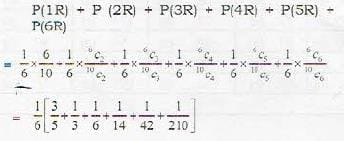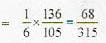Test: Probability and Statistics - 1 - Question 6

A six faced die is a biased one. it is thrice more likely to show an odd number than to show an even number. It is thrown twice. The probability that the sum of the numbers in the two throws is even, is.

Detailed Solution for Test: Probability and Statistics - 1 - Question 6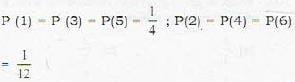Now sum of two odd numbers, and also sum of two even numbers is even, so.
P (odd, odd) + P (even, even)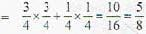Test: Probability and Statistics - 1 - Question 7

A letter is knwon to have come from either TATANAGAR' or CALCUTTA. On the envelope, Just two consecutive letters, TA, are visible. The probability that the letter has come from CALCUTTA is.

Detailed Solution for Test: Probability and Statistics - 1 - Question 7

TATANAGAR has 9 letters, so number of ways in which two consecutive letters can be printed will be, 8 out of which there are 2 ways in which TA can be printed.
Similarly for CALCUTTA, there are 7 ways of printing two consecutive letters, from which there is only way to print TA'
So, required probability’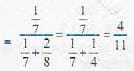Test: Probability and Statistics - 1 - Question 8

A card is drawn from a pack. The card is replaced and the pack is reshuffled. If this is done six times, the probability that 2 hearts, 2 diamonds and 2 club cards are drawn is.

Detailed Solution for Test: Probability and Statistics - 1 - Question 8

Probability of selecting card of any suit =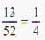so, due to replacement probability that out of 6 cards drawn there will be 2 Hearts, 2 diamonds and 2 club cards is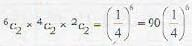Test: Probability and Statistics - 1 - Question 9

There are three piles of identical yellow, black and green balls and each pile contains at least 20 balls. The number of ways of selecting 20 balls if the number of black balls to be selected is twice the number of yellow balls is.

Detailed Solution for Test: Probability and Statistics - 1 - Question 9

Possible configuration of balls to be selected seven possible cases are there.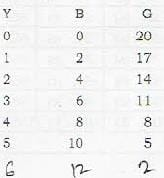Test: Probability and Statistics - 1 - Question 10

Two balls are drawn at random from a bag containing 6 white, 4 red and 5 black balls. The probability that both these balls are black, is :

Detailed Solution for Test: Probability and Statistics - 1 - Question 10

Required probability =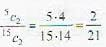Test: Probability and Statistics - 1 - Question 11

Let X be the random variable giving the number of heads obtained in 162 successive tosses of a biased coin with probability of getting head in a toss is 1/3.  Assume that the losses are in dependent. The standard deviation of X is.

Detailed Solution for Test: Probability and Statistics - 1 - Question 11

Random variable x, follows Binomial distribution with n = 162, p = 1/3so, q = 1 - p = 2/3
variance = npq = 162(1/3)(2/3) = 36
⇒ standard deviation = √variance = 6

Test: Probability and Statistics - 1 - Question 12

Assume that the number of buses arriving at a bus-stop in an interval of t minutes is poisson distributed with parameter 0.21. The probability that at least three buses will arrive during a 10 minute interval is.

Detailed Solution for Test: Probability and Statistics - 1 - Question 12

Probability mass function of poission distribution with parameter 0.2t is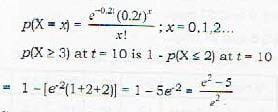Test: Probability and Statistics - 1 - Question 13

The standard deviation of first, n natural numbers is :

Detailed Solution for Test: Probability and Statistics - 1 - Question 13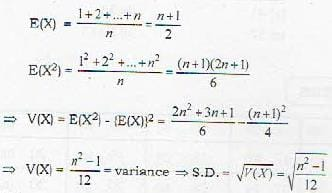Test: Probability and Statistics - 1 - Question 14

Of a distribution, coefficient of skewness is 0.32, standard deviation is 6.5 and the mean is 29.6 then its mode will be :

Detailed Solution for Test: Probability and Statistics - 1 - Question 14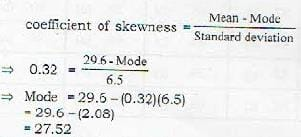Test: Probability and Statistics - 1 - Question 15

A ship arrives at a port and 40 sailors on board go ashore for revelry. Later at night, the 40 sailors return to the ship and in their state of inebriation, each chooses a random cabin to sleep in. What is the expected number of sailors sleeping in their own cabin?

Detailed Solution for Test: Probability and Statistics - 1 - Question 15

probability that the sailor returns in its own cabin is 1/40 If sailor is in his own cabin in  the expected value head count will be increased by 1.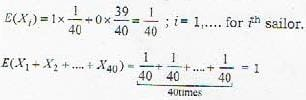Test: Probability and Statistics - 1 - Question 16

Suppose there are 15 different types of coupons and suppose that each time one obtains a coupon, it is equally likely to be any one of the 15 types. The expected number of different types that are contained in the set of 5 coupons is

Detailed Solution for Test: Probability and Statistics - 1 - Question 16

Probability that any particular coupon will be selected is 1/15.
Expected number of different types of coupons =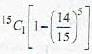Test: Probability and Statistics - 1 - Question 17

The joint density of X and Y is given by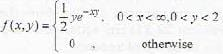The value of E|X/2|Y = 1 is equal to

Detailed Solution for Test: Probability and Statistics - 1 - Question 17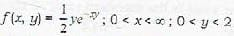Marginal density function of y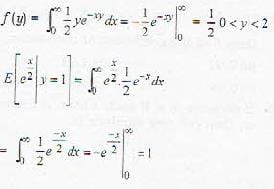Test: Probability and Statistics - 1 - Question 18

A and B throw with one die for a stake of Rs 11 which is to be won by the player who first throws 6. If A has the first throw, what is the expectation of his winning the stake?

Detailed Solution for Test: Probability and Statistics - 1 - Question 18

Probability of obtaining 6 in a single throw is 1/6. Probability that A wins the stake if he get 6 in first throw or if he loses then B also lose and then he get 6 in his second throw i.e. overal 3rd throw and so on.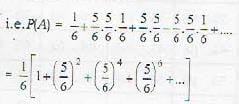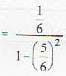[sum of infinite terms of G.P.]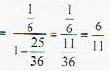Test: Probability and Statistics - 1 - Question 19

If the random variables X, Y and Z have the means μx = 2, μy= -3 and μz = 2, the variances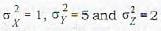and covariances cov(X,Y) = -2, cov(X, Z) = -1 and cov (Y, Z) = 1, the variance of W = 3X - Y+ 2Z is

Detailed Solution for Test: Probability and Statistics - 1 - Question 19

W = 3 X - Y + 2 Z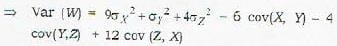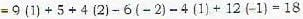Test: Probability and Statistics - 1 - Question 20

A computationally efficient way to compute the sample mean of the data x1,x2,...xn is as follows: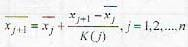Then K(j) is equal to

Detailed Solution for Test: Probability and Statistics - 1 - Question 20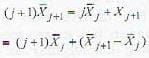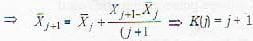## Topic-wise Tests & Solved Examples for IIT JAM Mathematics

27 docs|150 tests
 Use Code STAYHOME200 and get INR 200 additional OFF Use Coupon Code
Information about Test: Probability and Statistics - 1 Page
In this test you can find the Exam questions for Test: Probability and Statistics - 1 solved & explained in the simplest way possible. Besides giving Questions and answers for Test: Probability and Statistics - 1, EduRev gives you an ample number of Online tests for practice

## Topic-wise Tests & Solved Examples for IIT JAM Mathematics

27 docs|150 tests